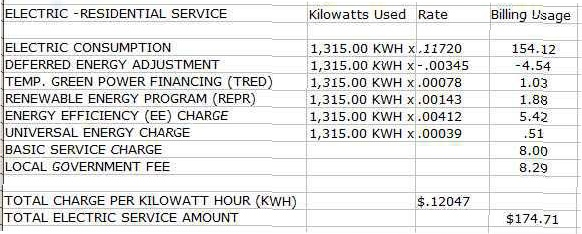﻿ Residential Electric Rates - Electrical 101# Residential Electric Rates

### How to Calculate the Electric Rate from Your Bill

Calculating the electric rate from your bill can be a little tricky. There are usually categories of charges and credits to determine your electric rate.

• Charges are added for different categories per kWh used.
• Credits are subtracted for different categories per kWh used.

In the example above, add the amounts in the “rate” column, but subtracting the “deferred energy adjustment” credit amount. The electric rate for this bill is \$.12047. Note the service charges are flat rates and are not affected by your energy usage. These charges are not included in your electric rate.

It is common for electric rates to change slightly over a short period of time. When using the energy usage calculators, calculate your exact rate for a given month, or use this “Average Electric Rates by State” link.

## How do I determine my electric rate?

• Check your power company’s website (or call customer service).
• Calculate electric rate from your power bill (add charges and subtract credits).
• Use the chart below for an approximate electric rate for your state.

Energy usage is measured in kilowatt hours (kWh). A kilowatt equals 1000 watts. A kilowatt hour equals 1000 watts for 1 hour.

Using 1 kWh of power at a rate of \$0.12 per kWh costs \$0.12.

Using 10 kWh of power at a rate of \$0.12 per kWh costs \$1.12.

## Residential Electric Rates by State

Average Electric Rates by State

### Sample Electric BillSave Energy

Standby (Phantom) Power

Energy Usage Calculators

Electric Meters

Residential Electric Rates